# Frank Solutions for Class 10 Maths Chapter 14 Symmetry

Frank Solutions for Class 10 Maths Chapter 14 Symmetry are provided here. In order to obtain a good academic score in Mathematics, the important thing to be done by the students is to solve the questions of each and every exercise. Frank Solutions for Class 10 Maths are prepared by a team of experts who work with their full potential to help the students excel in their exams. Step-by-step solutions to each question are given in simple, understandable language for the students to grasp concepts effortlessly.

Chapter 14 – Symmetry, a figure is said to have line symmetry, if, by folding the figure along a line, the left and right parts of it coincide exactly. The line is called the line (or axis) of the symmetry of the figure.

## Download the PDF of Frank Solutions for Class 10 Maths Chapter 14 Symmetry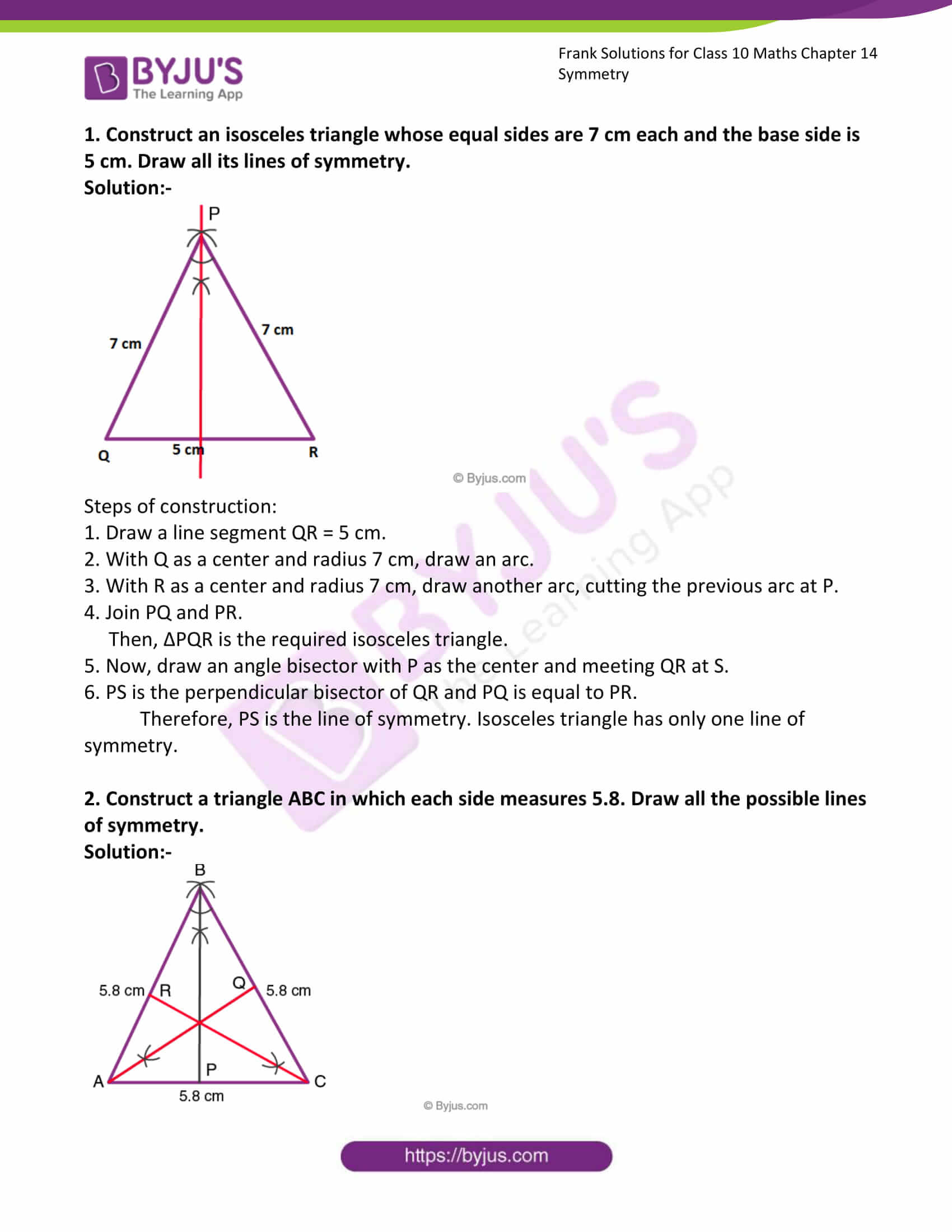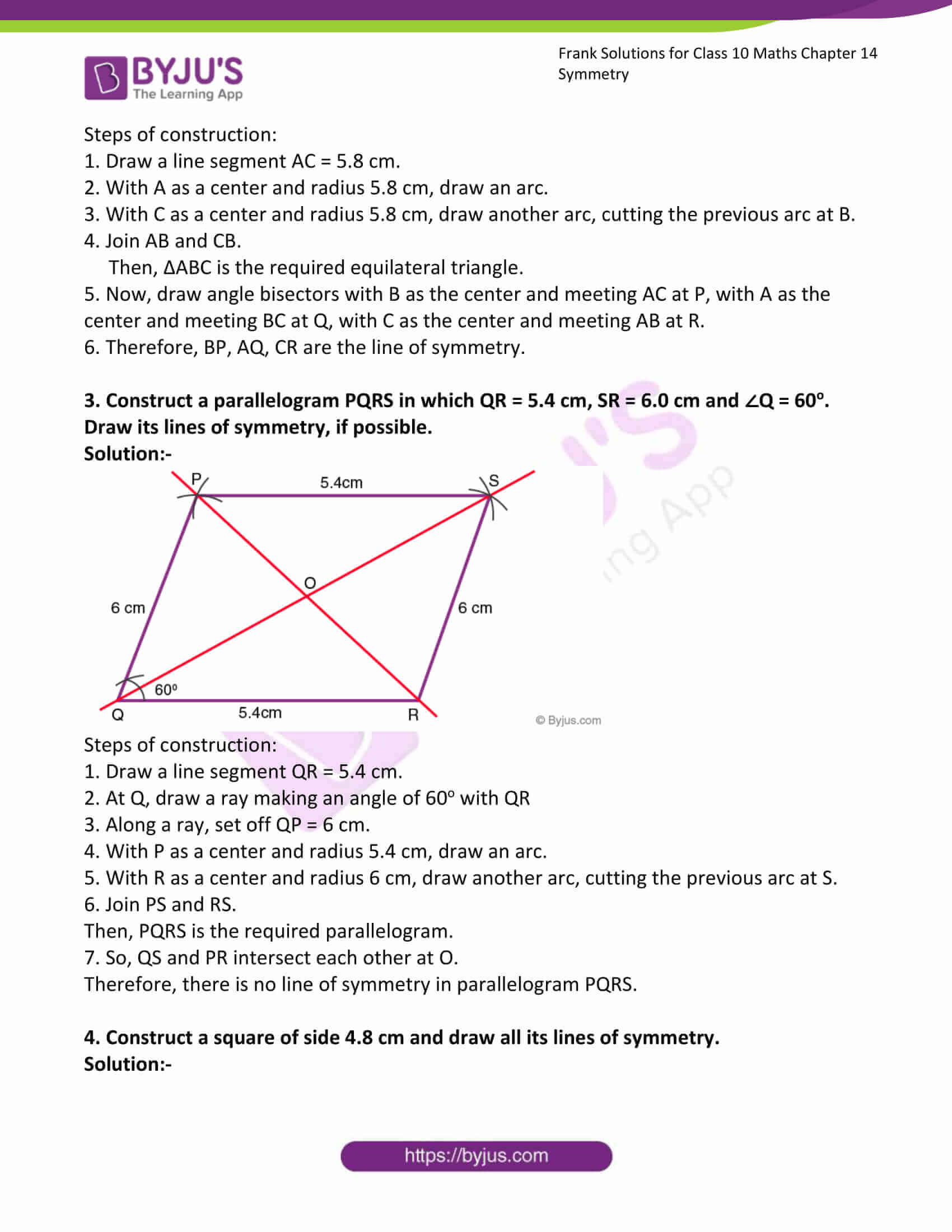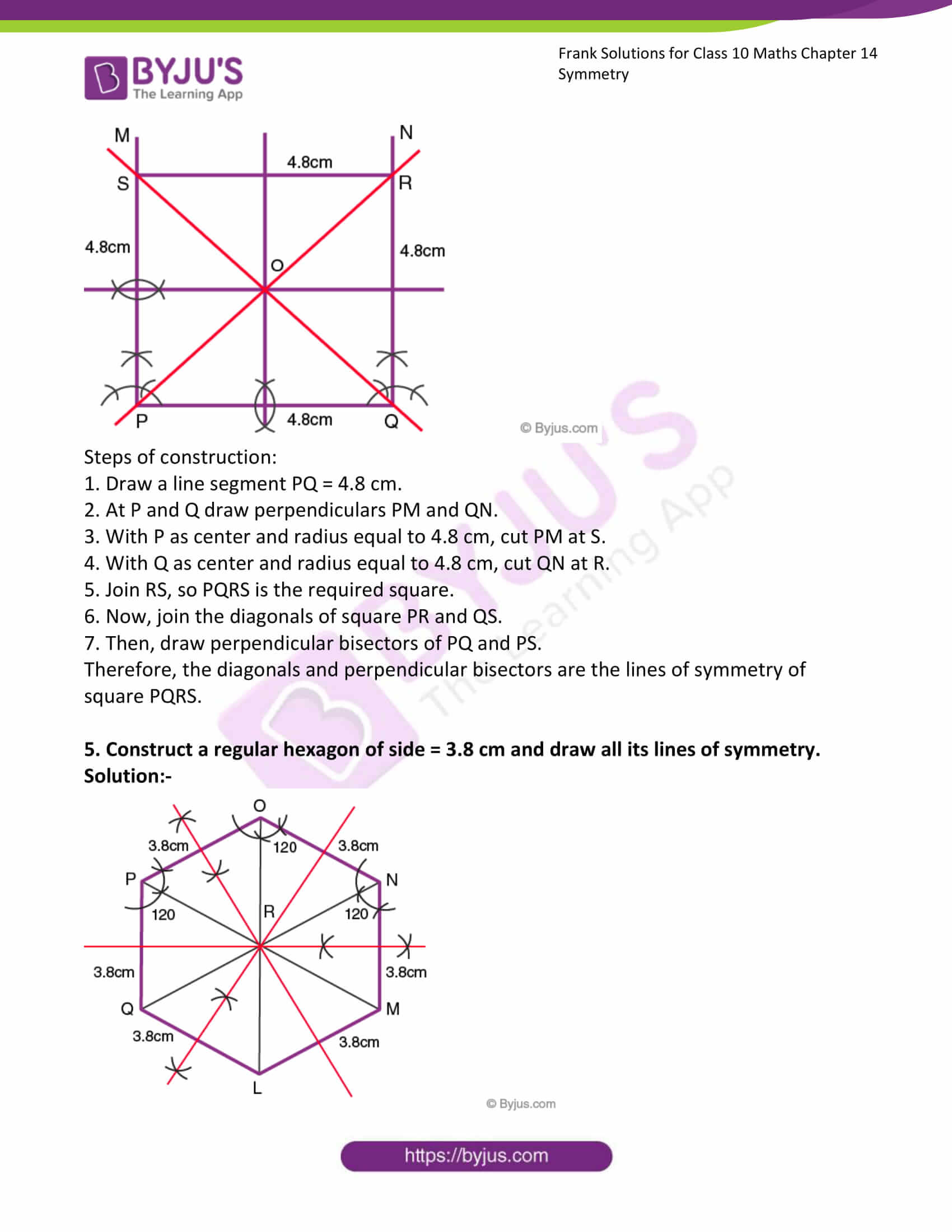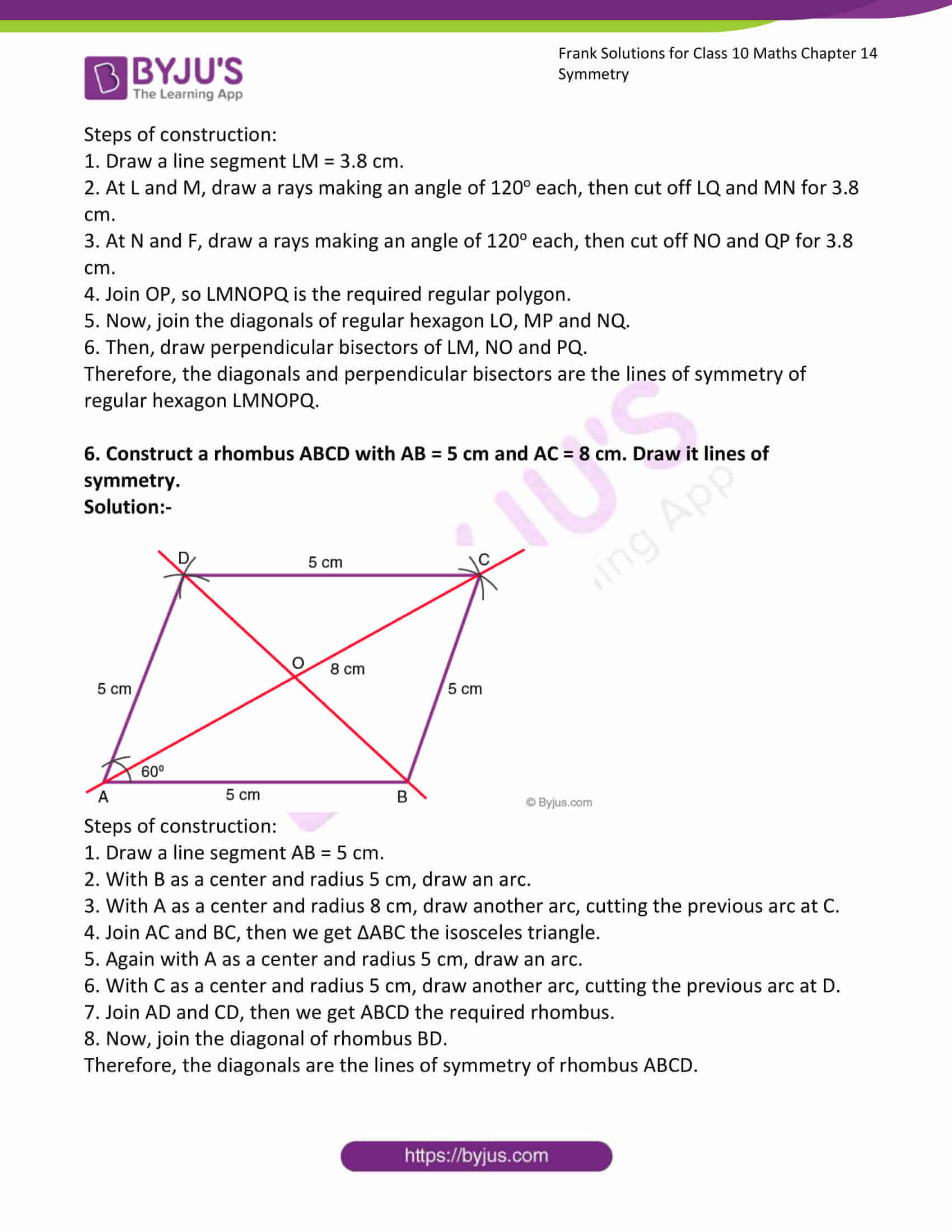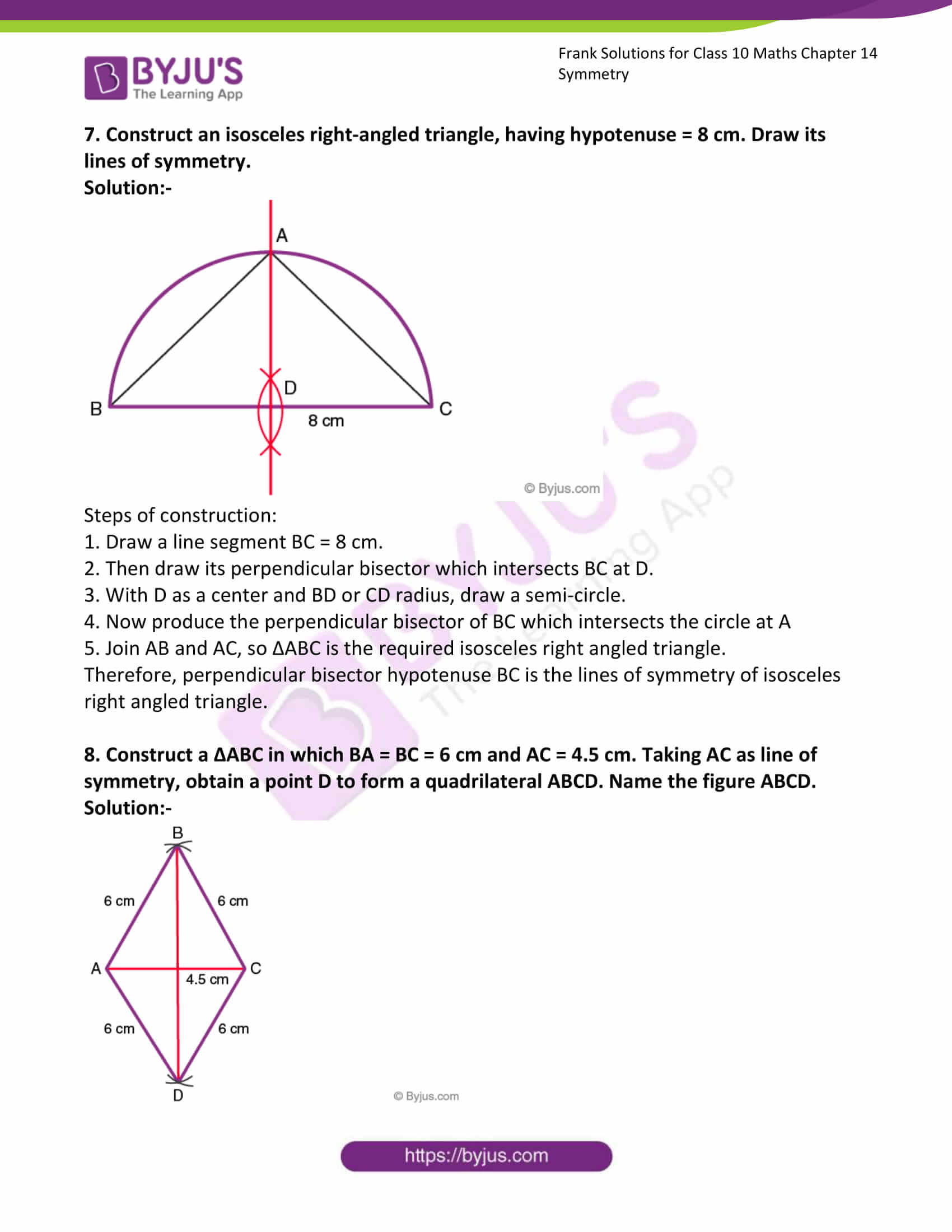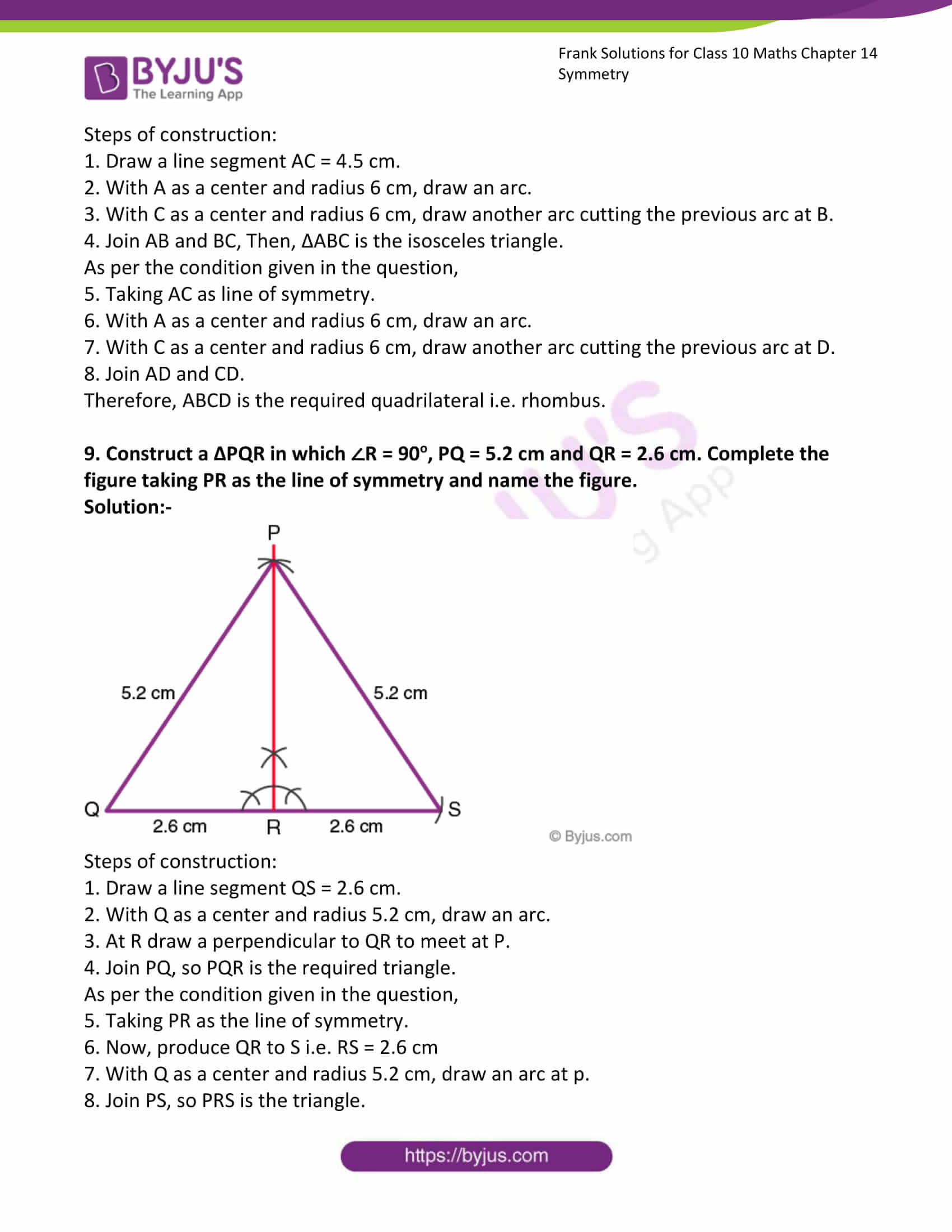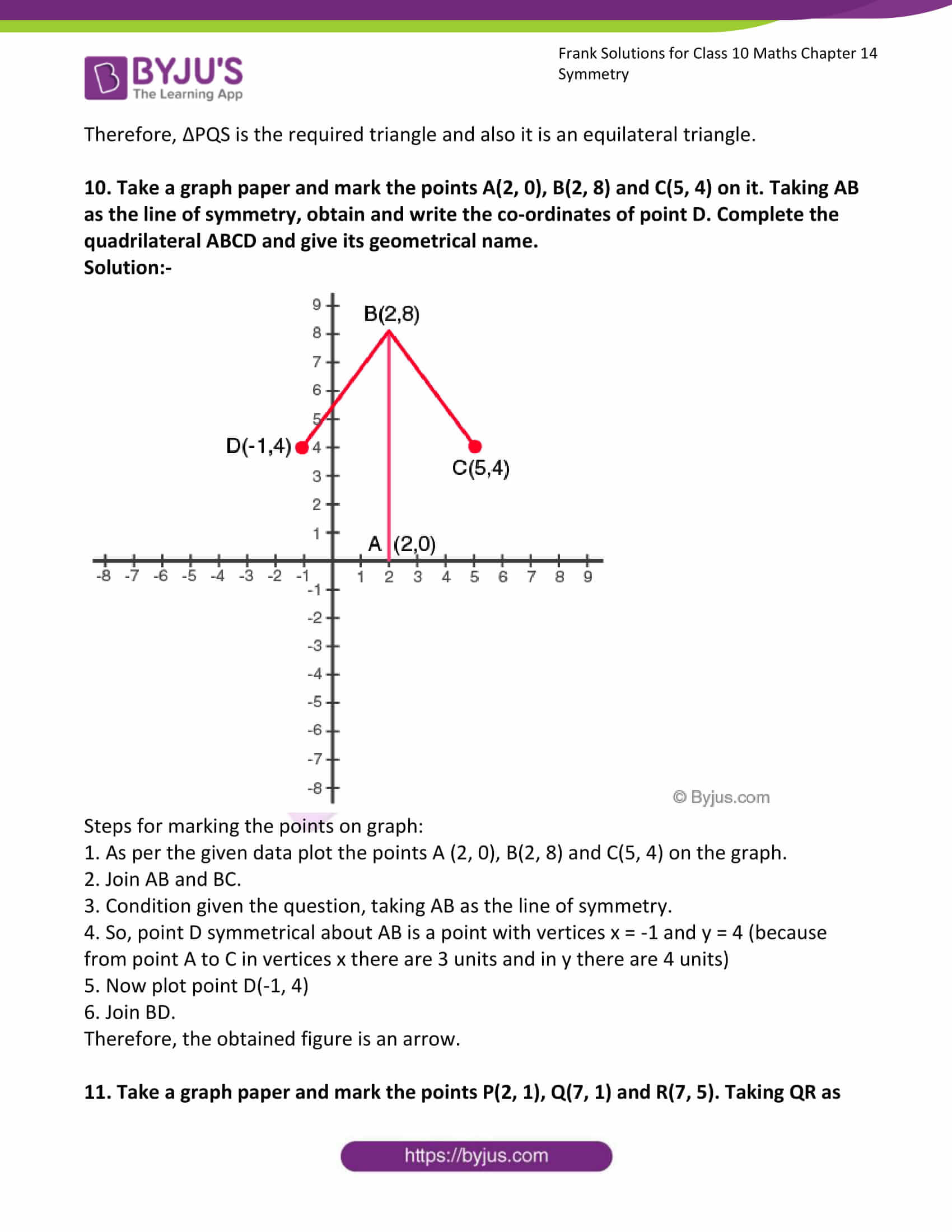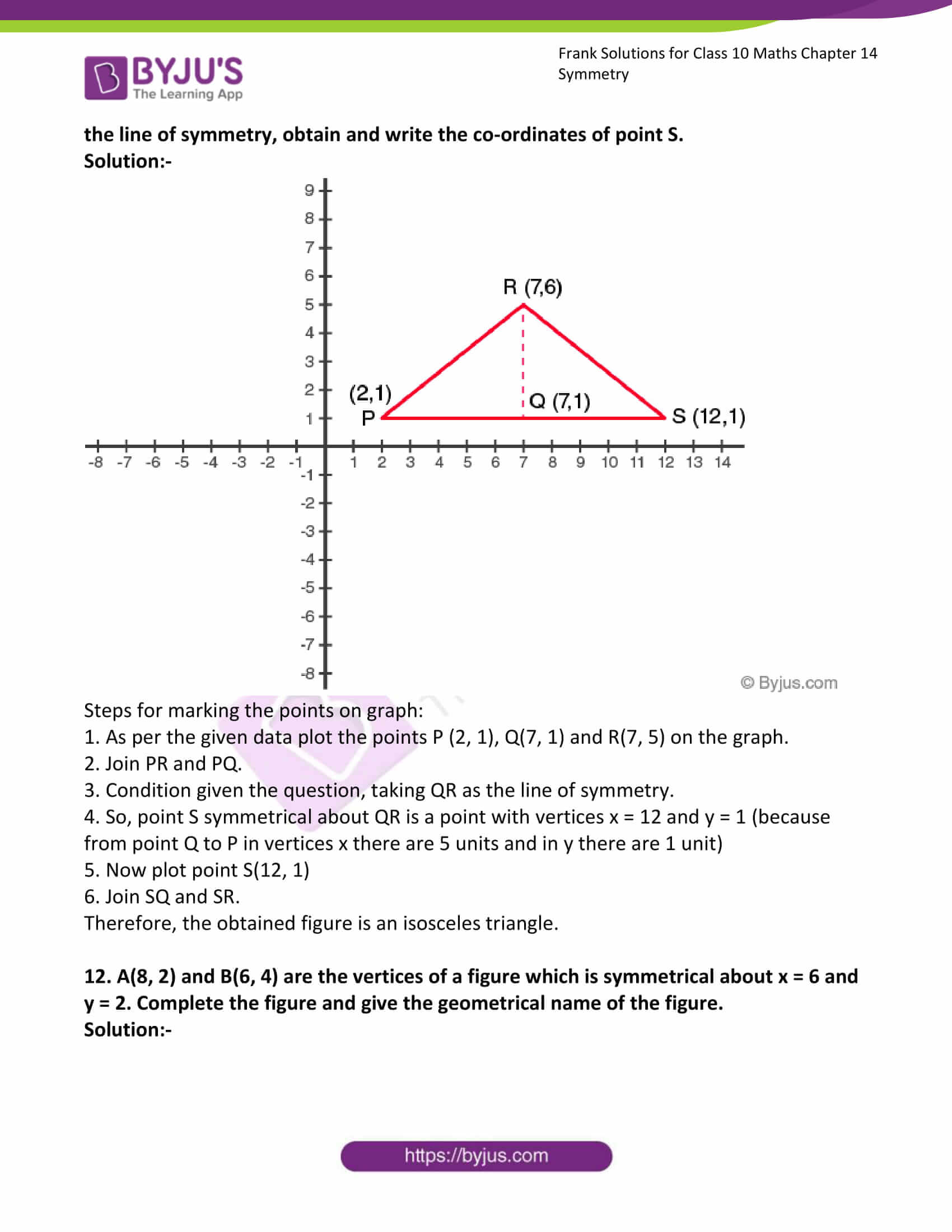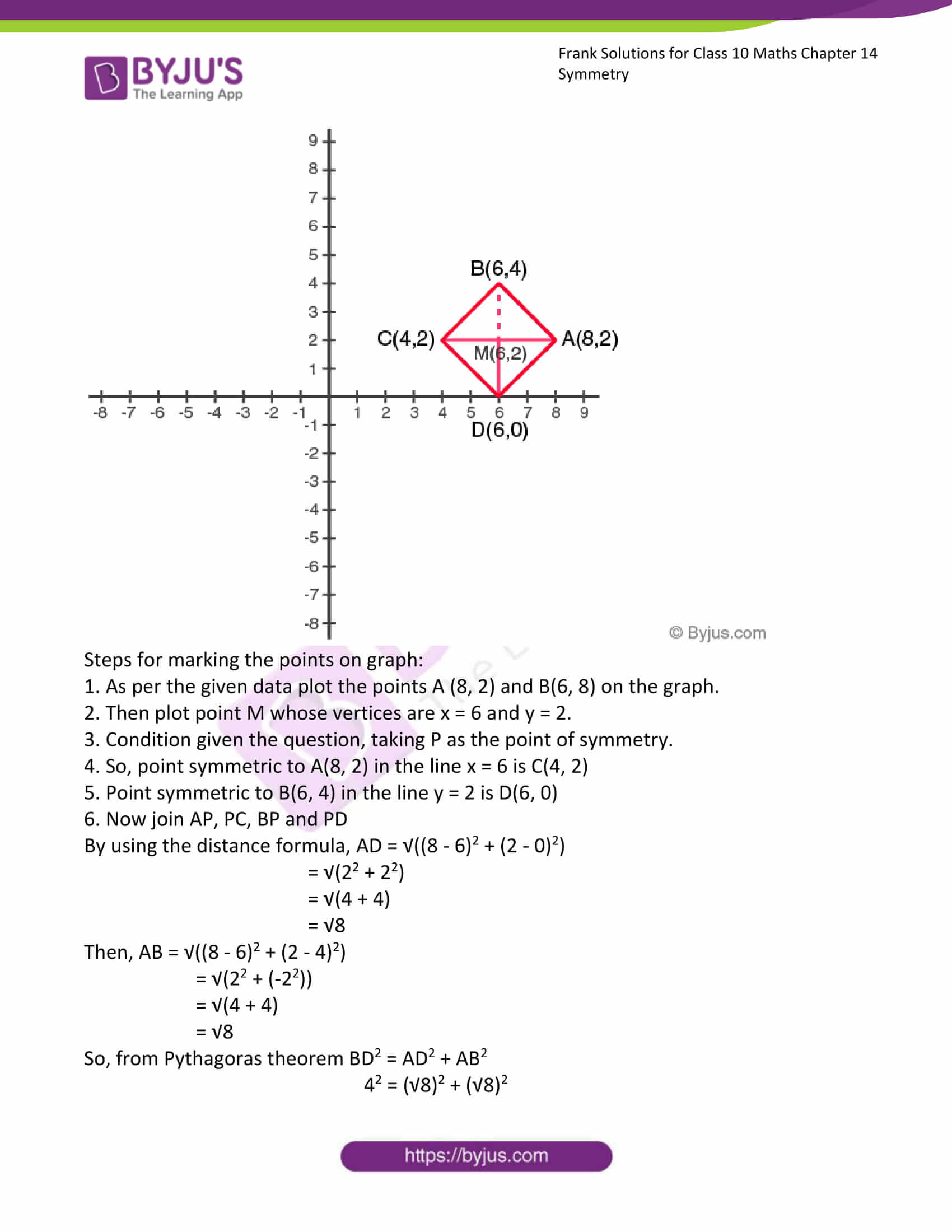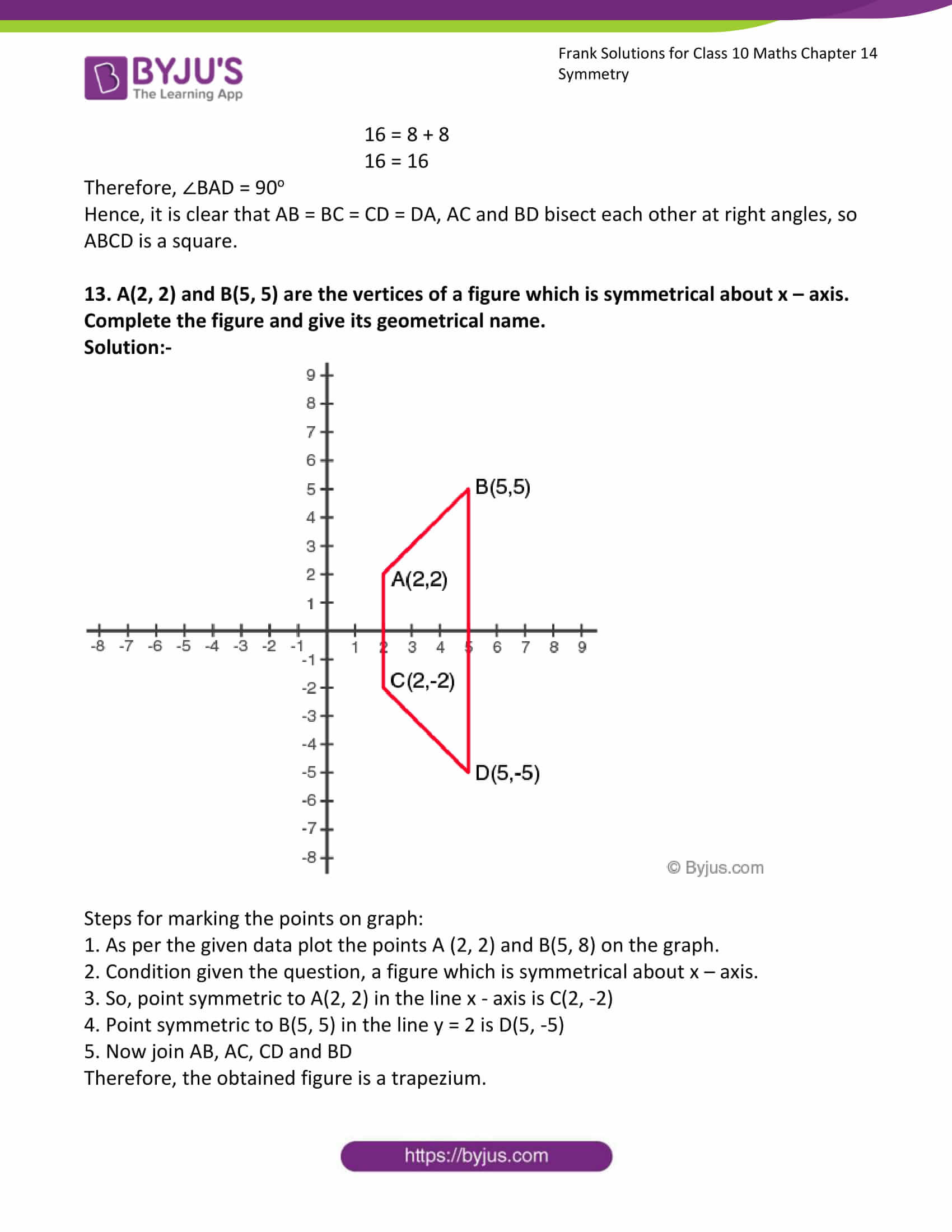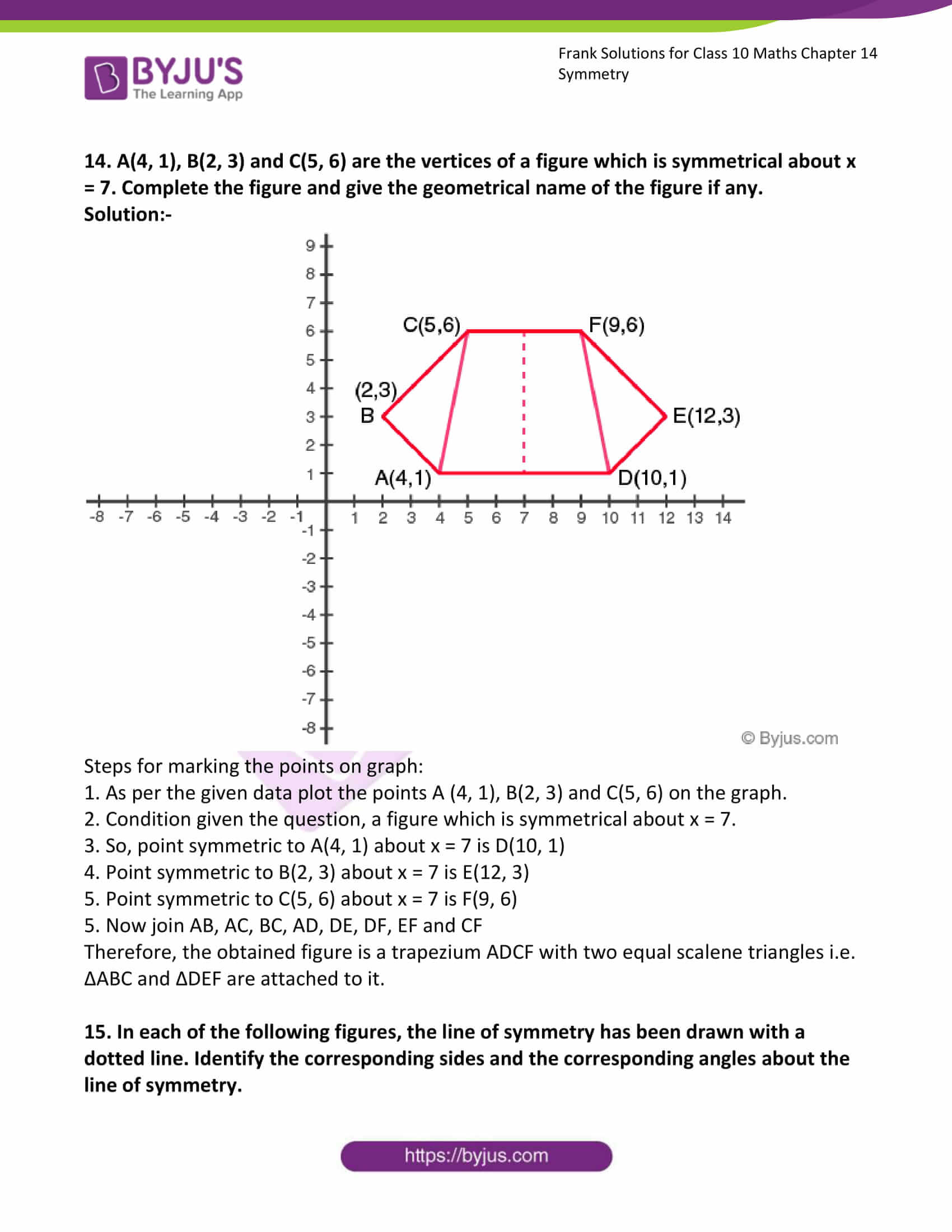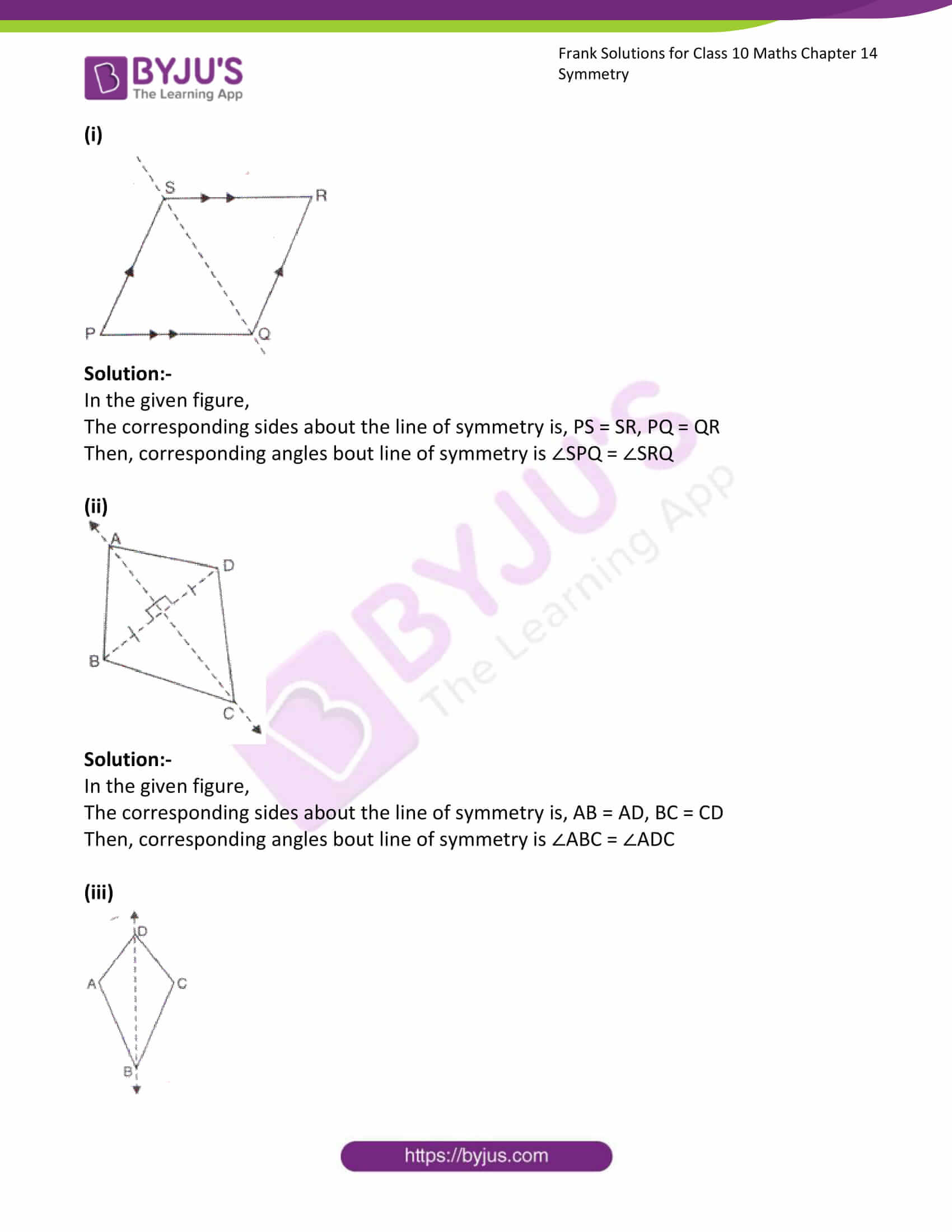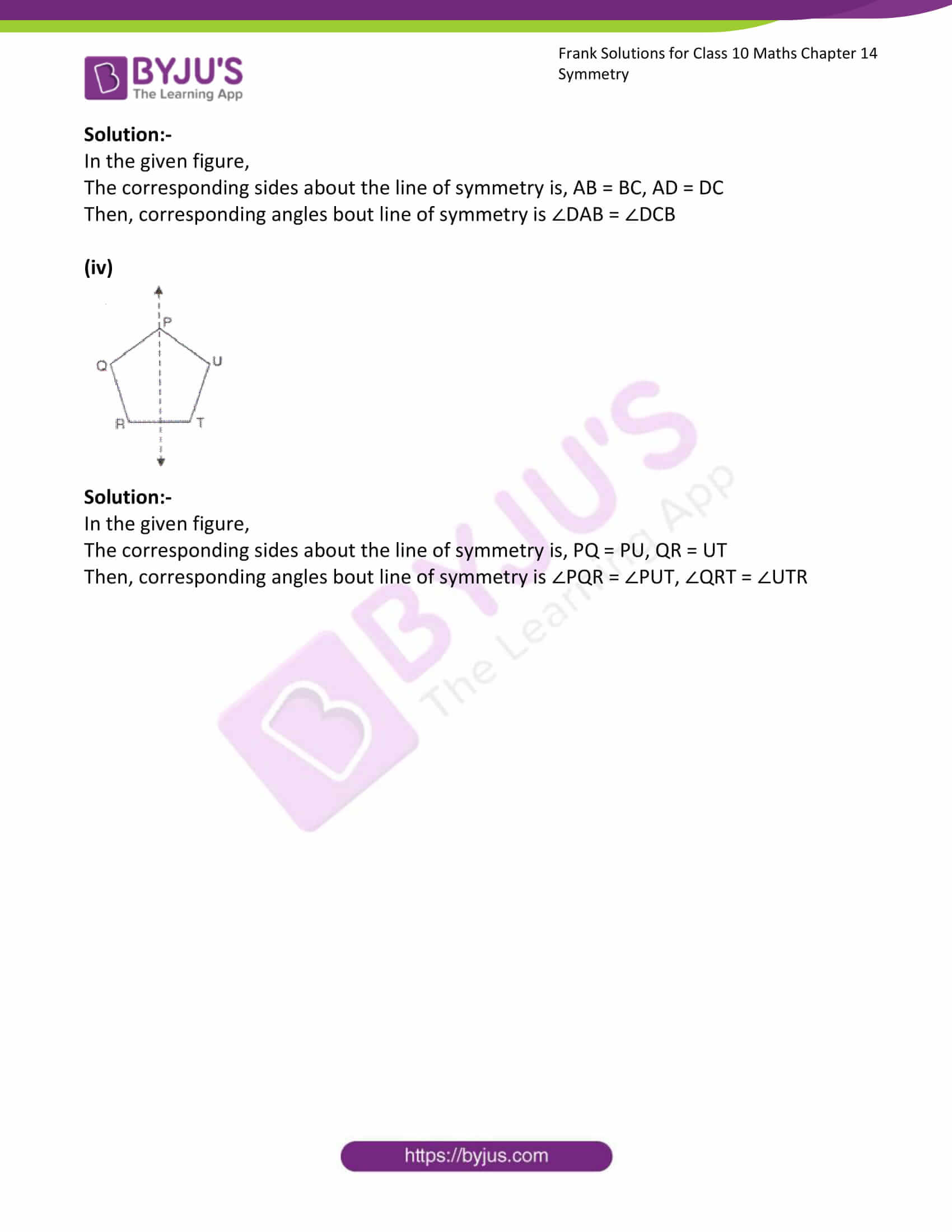### Access Answers to Frank Solutions for Class 10 Maths Chapter 14 Symmetry

1. Construct an isosceles triangle whose equal sides are 7 cm each and the base side is 5 cm. Draw all its lines of symmetry.

Solution:-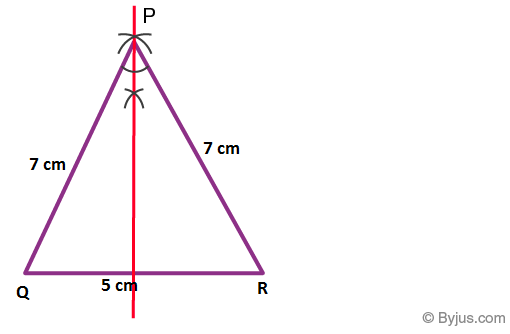Steps of construction:

1. Draw a line segment QR = 5 cm.

2. With Q as a centre and radius 7 cm, draw an arc.

3. With R as a centre and radius 7 cm, draw another arc, cutting the previous arc at P.

4. Join PQ and PR.

Then, ΔPQR is the required isosceles triangle.

5. Now, draw an angle bisector with P as the centre and meeting QR at S.

6. PS is the perpendicular bisector of QR, and PQ is equal to PR.

Therefore, PS is the line of symmetry. Isosceles triangle has only one line of symmetry.

2. Construct a triangle ABC in which each side measures 5.8. Draw all the possible lines of symmetry.

Solution:-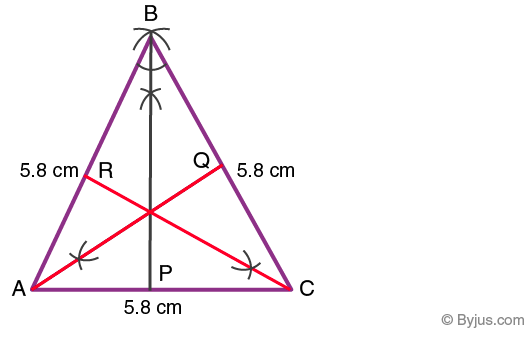Steps of construction:

1. Draw a line segment AC = 5.8 cm.

2. With A as a centre and radius 5.8 cm, draw an arc.

3. With C as a centre and radius 5.8 cm, draw another arc, cutting the previous arc at B.

4. Join AB and CB.

Then, ΔABC is the required equilateral triangle.

5. Now, draw angle bisectors with B as the centre and meeting AC at P, with A as the centre and meeting BC at Q, with C as the centre and meeting AB at R.

6. Therefore, BP, AQ, CR are the line of symmetry.

3. Construct a parallelogram PQRS in which QR = 5.4 cm, SR = 6.0 cm and ∠Q = 60o. Draw its lines of symmetry, if possible.

Solution:-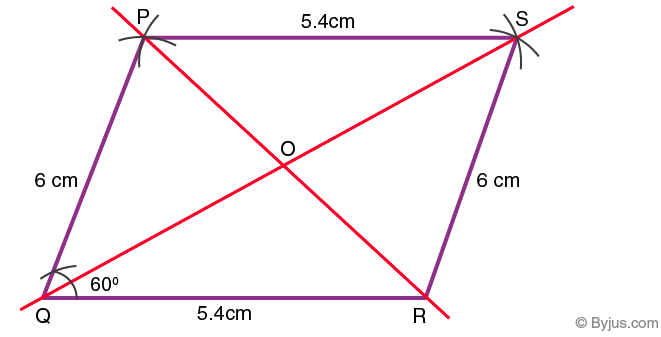Steps of construction:

1. Draw a line segment QR = 5.4 cm.

2. At Q, draw a ray making an angle of 60o with QR

3. Along a ray, set off QP = 6 cm.

4. With P as a centre and radius 5.4 cm, draw an arc.

5. With R as a centre and radius 6 cm, draw another arc, cutting the previous arc at S.

6. Join PS and RS.

Then, PQRS is the required parallelogram.

7. So, QS and PR intersect each other at O.

Therefore, there is no line of symmetry in parallelogram PQRS.

4. Construct a square of side 4.8 cm and draw all its lines of symmetry.

Solution:-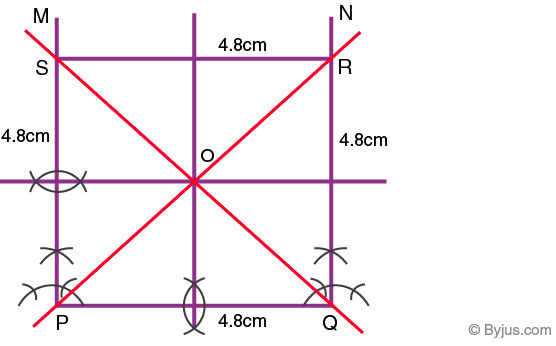Steps of construction:

1. Draw a line segment PQ = 4.8 cm.

2. At P and Q draw perpendiculars PM and QN.

3. With P as centre and radius equal to 4.8 cm, cut PM at S.

4. With Q as centre and radius equal to 4.8 cm, cut QN at R.

5. Join RS, so PQRS is the required square.

6. Now, join the diagonals of square PR and QS.

7. Then, draw perpendicular bisectors of PQ and PS.

Therefore, the diagonals and perpendicular bisectors are the lines of symmetry of square PQRS.

5. Construct a regular hexagon of side = 3.8 cm and draw all its lines of symmetry.

Solution:-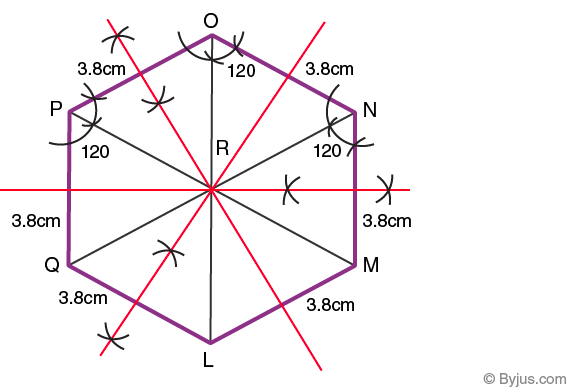Steps of construction:

1. Draw a line segment LM = 3.8 cm.

2. At L and M, draw a rays making an angle of 120o each, then cut off LQ and MN for 3.8 cm.

3. At N and F, draw a rays making an angle of 120o each, then cut off NO and QP for 3.8 cm.

4. Join OP, so LMNOPQ is the required regular polygon.

5. Now, join the diagonals of regular hexagon LO, MP and NQ.

6. Then, draw perpendicular bisectors of LM, NO and PQ.

Therefore, the diagonals and perpendicular bisectors are the lines of symmetry of regular hexagon LMNOPQ.

6. Construct a rhombus ABCD with AB = 5 cm and AC = 8 cm. Draw it lines of symmetry.

Solution:-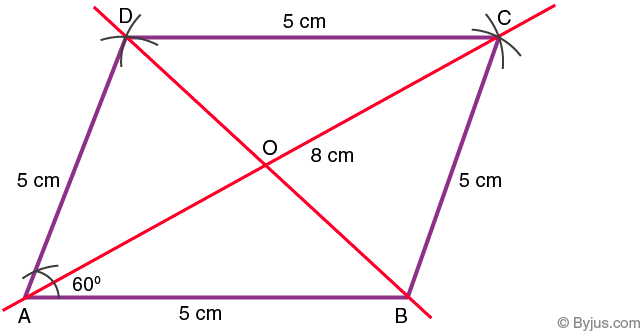Steps of construction:

1. Draw a line segment AB = 5 cm.

2. With B as a centre and radius 5 cm, draw an arc.

3. With A as a centre and radius 8 cm, draw another arc, cutting the previous arc at C.

4. Join AC and BC, then we get ΔABC the isosceles triangle.

5. Again with A as a centre and radius 5 cm, draw an arc.

6. With C as a centre and radius 5 cm, draw another arc, cutting the previous arc at D.

7. Join AD and CD, then we get ABCD the required rhombus.

8. Now, join the diagonal of rhombus BD.

Therefore, the diagonals are the lines of symmetry of rhombus ABCD.

7. Construct an isosceles right-angled triangle, having hypotenuse = 8 cm. Draw its lines of symmetry.

Solution:-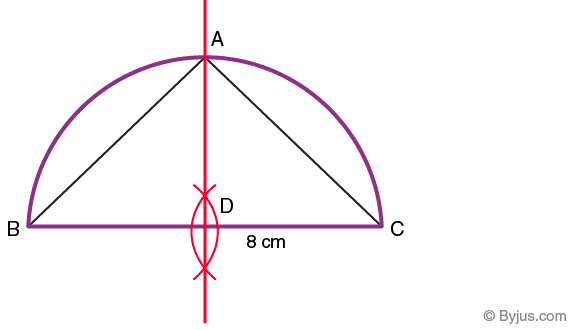Steps of construction:

1. Draw a line segment BC = 8 cm.

2. Then draw its perpendicular bisector, which intersects BC at D.

3. With D as a centre and BD or CD radius, draw a semi-circle.

4. Now produce the perpendicular bisector of BC, which intersects the circle at A

5. Join AB and AC, so ΔABC is the required isosceles right angled triangle.

Therefore, perpendicular bisector hypotenuse BC is the lines of symmetry of isosceles right angled triangle.

8. Construct a ΔABC in which BA = BC = 6 cm and AC = 4.5 cm. Taking AC as line of symmetry, obtain a point D to form a quadrilateral ABCD. Name the figure ABCD.

Solution:-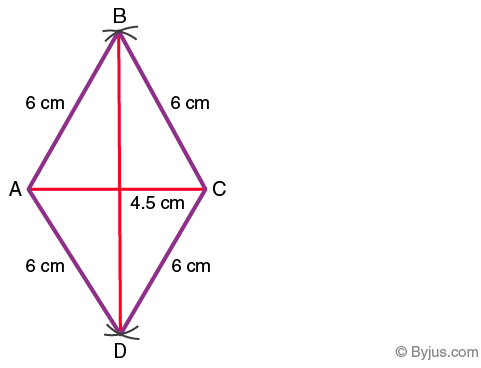Steps of construction:

1. Draw a line segment AC = 4.5 cm.

2. With A as a centre and radius 6 cm, draw an arc.

3. With C as a centre and radius 6 cm, draw another arc cutting the previous arc at B.

4. Join AB and BC, Then, ΔABC is the isosceles triangle.

As per the condition given in the question,

5. Taking AC as line of symmetry.

6. With A as a centre and radius 6 cm, draw an arc.

7. With C as a centre and radius 6 cm, draw another arc cutting the previous arc at D.

Therefore, ABCD is the required quadrilateral i.e. rhombus.

9. Construct a ΔPQR in which ∠R = 90o, PQ = 5.2 cm and QR = 2.6 cm. Complete the figure taking PR as the line of symmetry and name the figure.

Solution:-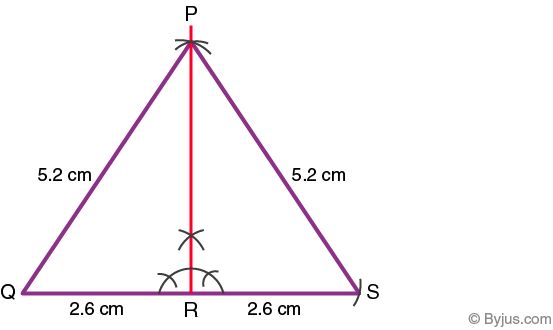Steps of construction:

1. Draw a line segment QS = 2.6 cm.

2. With Q as a centre and radius 5.2 cm, draw an arc.

3. At R draw a perpendicular to QR to meet at P.

4. Join PQ, so PQR is the required triangle.

As per the condition given in the question,

5. Taking PR as the line of symmetry.

6. Now, produce QR to S i.e. RS = 2.6 cm

7. With Q as a centre and radius 5.2 cm, draw an arc at p.

8. Join PS, so PRS is the triangle.

Therefore, ΔPQS is the required triangle, and also it is an equilateral triangle.

10. Take a graph paper and mark the points A(2, 0), B(2, 8) and C(5, 4) on it. Taking AB as the line of symmetry, obtain and write the co-ordinates of point D. Complete the quadrilateral ABCD and give its geometrical name.

Solution:-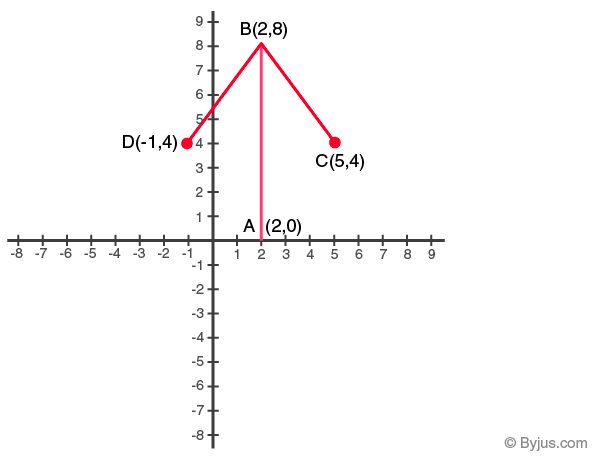Steps for marking the points on graph:

1. As per the given data plot the points A (2, 0), B(2, 8) and C(5, 4) on the graph.

2. Join AB and BC.

3. Condition given the question, taking AB as the line of symmetry.

4. So, point D symmetrical about AB is a point with vertices x = -1 and y = 4 (because from point A to C in vertices x there are 3 units and in y there are 4 units)

5. Now plot point D(-1, 4)

6. Join BD.

Therefore, the obtained figure is an arrow.

11. Take a graph paper and mark the points P(2, 1), Q(7, 1) and R(7, 5). Taking QR as the line of symmetry, obtain and write the co-ordinates of point S.

Solution:-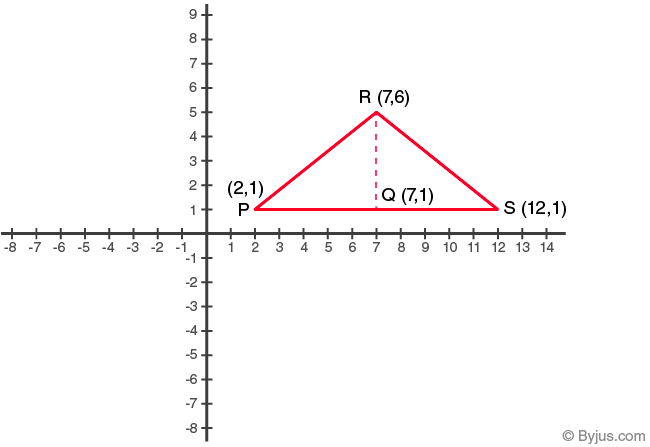Steps for marking the points on graph:

1. As per the given data plot the points P (2, 1), Q(7, 1) and R(7, 5) on the graph.

2. Join PR and PQ.

3. Condition given the question, taking QR as the line of symmetry.

4. So, point S symmetrical about QR is a point with vertices x = 12 and y = 1 (because from point Q to P in vertices x there are 5 units and in y there are 1 unit)

5. Now plot point S(12, 1)

6. Join SQ and SR.

Therefore, the obtained figure is an isosceles triangle.

12. A(8, 2) and B(6, 4) are the vertices of a figure which is symmetrical about x = 6 and y = 2. Complete the figure and give the geometrical name of the figure.

Solution:-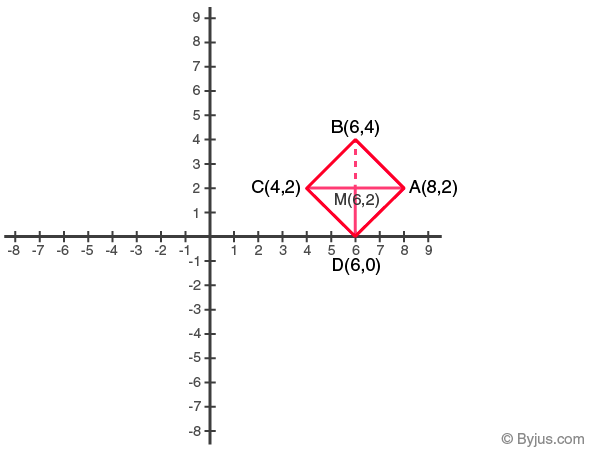Steps for marking the points on graph:

1. As per the given data plot the points A (8, 2) and B(6, 8) on the graph.

2. Then plot point M whose vertices are x = 6 and y = 2.

3. Condition given the question, taking P as the point of symmetry.

4. So, point symmetric to A(8, 2) in the line x = 6 is C(4, 2)

5. Point symmetric to B(6, 4) in the line y = 2 is D(6, 0)

6. Now join AP, PC, BP and PD

By using the distance formula, AD = √((8 – 6)2 + (2 – 0)2)

= √(22 + 22)

= √(4 + 4)

= √8

Then, AB = √((8 – 6)2 + (2 – 4)2)

= √(22 + (-22))

= √(4 + 4)

= √8

So, from Pythagoras theorem BD2 = AD2 + AB2

42 = (√8)2 + (√8)2

16 = 8 + 8

16 = 16

Hence, it is clear that AB = BC = CD = DA, AC and BD bisect each other at right angles, so ABCD is a square.

13. A(2, 2) and B(5, 5) are the vertices of a figure which is symmetrical about x – axis. Complete the figure and give its geometrical name.

Solution:-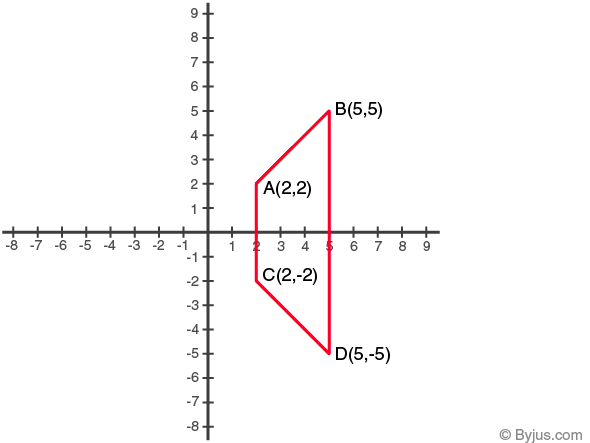Steps for marking the points on graph:

1. As per the given data plot the points A (2, 2) and B(5, 8) on the graph.

2. Condition given the question, a figure which is symmetrical about x – axis.

3. So, point symmetric to A(2, 2) in the line x – axis is C(2, -2)

4. Point symmetric to B(5, 5) in the line y = 2 is D(5, -5)

5. Now join AB, AC, CD and BD

Therefore, the obtained figure is a trapezium.

14. A(4, 1), B(2, 3) and C(5, 6) are the vertices of a figure which is symmetrical about x = 7. Complete the figure and give the geometrical name of the figure if any.

Solution:-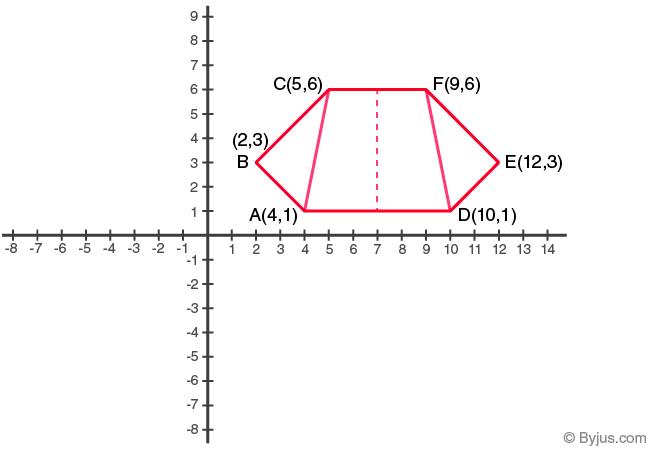Steps for marking the points on graph:

1. As per the given data plot the points A (4, 1), B(2, 3) and C(5, 6) on the graph.

2. Condition given the question, a figure which is symmetrical about x = 7.

3. So, point symmetric to A(4, 1) about x = 7 is D(10, 1)

4. Point symmetric to B(2, 3) about x = 7 is E(12, 3)

5. Point symmetric to C(5, 6) about x = 7 is F(9, 6)

5. Now join AB, AC, BC, AD, DE, DF, EF and CF

Therefore, the obtained figure is a trapezium ADCF with two equal scalene triangles i.e. ΔABC and ΔDEF are attached to it.

15. In each of the following figures, the line of symmetry has been drawn with a dotted line. Identify the corresponding sides and the corresponding angles about the line of symmetry.

(i)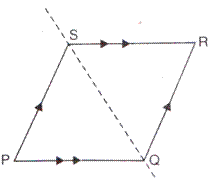Solution:-

In the given figure,

The corresponding sides about the line of symmetry is, PS = SR, PQ = QR

Then, corresponding angles bout line of symmetry is ∠SPQ = ∠SRQ

(ii)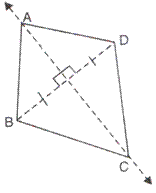Solution:-

In the given figure,

The corresponding sides about the line of symmetry is, AB = AD, BC = CD

Then, corresponding angles bout line of symmetry is ∠ABC = ∠ADC

(iii)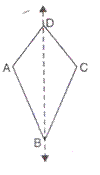Solution:-

In the given figure,

The corresponding sides about the line of symmetry is, AB = BC, AD = DC

Then, corresponding angles bout line of symmetry is ∠DAB = ∠DCB

(iv)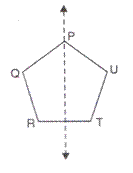Solution:-

In the given figure,

The corresponding sides about the line of symmetry is, PQ = PU, QR = UT

Then, corresponding angles bout line of symmetry is ∠PQR = ∠PUT, ∠QRT = ∠UTR